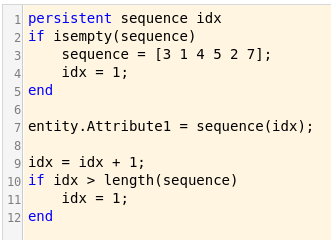## Write Event Actions for Legacy Models

When migrating legacy SimEvents® models, you often must create event actions in these instances:

• Setting attribute values

• Getting attribute values

• Generating random number generation

• Using Event sequences

• Replacing Attribute Function blocks

• Using Simulink® signals in an event-based computation

### Replace Set Attribute Blocks with Event Actions

Use these guidelines to replace Set Attribute blocks:

• If the Set Attribute blocks immediately follow entity generator blocks to initialize attributes, in the Entity Generator block, code the `Generate` action on the Event actions tab to set the attribute initial value. For example:

`entitySys.id=5;`
• If the Set Attribute blocks change attributes, in the Entity Generator block, code the `Create` action on the Event actions tab.

This example illustrates the `Generation` action to initialize the attribute values:### Get Attribute Values

If you write event actions to get attribute values, use a Simulink Function block:

1. Place the computation block in a Simulink Function block.

2. Pass the attribute value as an argument from the event action to the Simulink Function block.

### Replace Random Number Distributions in Event Actions

You can generate random numbers using:

#### Random Number Distribution

Replace Event-Based Random Number block random number distribution modes with equivalent MATLAB® code in event actions. For more information about generating random distributions, see Event Action Languages and Random Number Generation.

If you need additional random number distributions, see Statistics and Machine Learning Toolbox.

#### Example of Arbitrary Discrete Distribution Replacement

Here is an example of how to reproduce the arbitrary discrete distribution for the Event-Based Random Number legacy block. Assume that the block has these parameter settings:

• Distribution: ```Arbitrary discrete```

• Value vector: `[2 3 4 5 6]`

• Probability vector: `[0.3 0.3 0.1 0.2 0.1]`

• Initial seed: `12234`

As a general guideline:

1. Set the initial seed, for example:

```persistent init if isempty(init) rng(12234); init=true; end```
2. Determine what the value vector is assigned to in the legacy model and directly assign it in the action code in the new model. In this example, the value vector is assigned to the `FinalStop`.

3. To assign values within the appropriate range, calculate the cumulative probability vector. For convenience, use the probability vector to calculate the cumulative probably vector. For example, if the probability vector is:

`[0.3 0.3 0.1 0.2 0.1]`

The cumulative probability vector is:

`[0.3 0.6 0.7 0.9 1]`
4. Create a random variable to use in the code, for example:

`x=rand();`

Here is example code for this example block to calculate the distribution. The value vector is assigned to `FinalStop`:

```% Set initial seed. persistent init if isempty(init) rng(12234); init=true; end % Create random variable, x. x=rand(); % % Assign values within the appropriate range using the cumulative probability vector. % if x < 0.3 entity.FinalStop=2; elseif x >= 0.3 && x< 0.6 entity.FinalStop=3; elseif x >= 0.6 && x< 0.7 entity.FinalStop=4; elseif x >= 0.7 && x< 0.9 entity.FinalStop=5; else entity.FinalStop=6; end```

### Replace Event-Based Sequence Block with Event Actions

Replace Event-Based Sequence blocks, which generate a sequence of numbers from specified column vectors, with event actions:### Replace Attribute Function Blocks with Event Actions

Replace Attribute Function blocks, which manipulate attributes using MATLAB code, with event actions:

1. Copy the Attribute Function code, without the function syntax, to the Event actions tab in the relevant event action.

2. To refer to the entity attribute, use the format `entity.Attribute1`.

For short or simple code, use constructs like these:If you have longer or more complicated code, consider replacing the Attribute Function block with a Simulink Function and copying the code without modification into the Simulink Function block.### If Using Simulink Signals in an Event-Based Computation

If you are using Simulink signals in an event-based computation, send the signals to a Simulink Function block.

1. Copy the event-based computation code to a Simulink Function block.

2. Send the Simulink signals as inputs to the Simulink Function block.

For example: